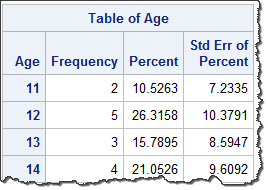## Generating SE with Proc Freq

I am trying to get Standard error for percentage for different levels of categorical variables (such as race) by using proc freq.

I've had no difficulty getting that with proc means but nothing seems to be working with Proc Freq.

Any help would be appreciated!

Thanks,

Kiran

1 ACCEPTED SOLUTION

Accepted Solutions

## Re: Generating SE with Proc Freq

I'm beyond my depth here, statistically speaking.  But syntax-wise, I know that PROC SURVEYFREQ calculates Standard Error of Percentage out of the box:

``````proc surveyfreq data=sashelp.class;
tables age ;
run;``````8 REPLIES 8

## Re: Generating SE with Proc Freq

The standard error of a percentage is

sqrt (p * (1 - p) / n)

--
Paige Miller

## Re: Generating SE with Proc Freq

I've had no difficulty getting that with proc means but nothing seems to be working with Proc Freq.

What did you try?

## Re: Generating SE with Proc Freq

I have tried the following statements:

proc freq data = merged12 STDERR;
tables ATTOBEVR;run;

proc freq data = merged12;
tables ATTOBEVR / cl; run;

proc freq data = merged12;
tables ATTOBEVR / std; run;

## Re: Generating SE with Proc Freq

There is no STD option in PROC FREQ. The CL option does not apply to percentage. The formula I posted earlier provides the proper way to get standard errors of percentages.

--
Paige Miller

## Re: Generating SE with Proc Freq

I'm beyond my depth here, statistically speaking.  But syntax-wise, I know that PROC SURVEYFREQ calculates Standard Error of Percentage out of the box:

``````proc surveyfreq data=sashelp.class;
tables age ;
run;``````## Re: Generating SE with Proc Freq

I don't think those are the same thing, because those take into account the survey design somehow. At least, they don't match the calculations of the formula for standard error of a percent in the non-survey case.

--
Paige Miller

## Re: Generating SE with Proc Freq

So it's a one way table. So you can calculate the percentages and the CI for them using the BINOMIAL option. Not sure how this works with more than two levels so make sure you test it.

``````proc freq data=sashelp.class ;
table sex / binomial (level='F'); *specifies Cl and boundaries for F;
run;``````

Since this is one level at a time it's definitely easier to do the hand calculations shown by @PaigeMiller

``````/*This program calculates binomial percentages and confidence limits.
Howver, this has no correction methodology so the lower bound can go below 0.
In this case a different method is needed*/

*set table name to summarize;
%let dsin=sashelp.class;
*set variable name to get percentages;
%let var = sex;
*set name of output data set;
%let dsout = Want;

*get counts of each by group and the total count;
proc sql noprint;
create table _freq as
select &var., count(*) as N
from &dsin
group by &var.;
select count(*) into :Ntotal from &dsin.;
quit;

*calculate percentages and upper/lower confidence limits;
data &dsout.;
set _freq;

Ntotal=&ntotal.;
PCT = n/Ntotal;

STD = ((PCT*(1-PCT))/NTotal)**(1/2);

UL = PCT + 1.96*STD;
LL = PCT - 1.96*STD;

run;

*remove temporary tables;
proc sql noprint;
drop table _freq;
quit;
``````

## Re: Generating SE with Proc Freq

This might be more information than you want, but @FreelanceReinh previously wrote an excellent answer to a related question and provided a program that computes standard errors of multinomial proportions and shows how to get the proportions, std errs, and CIs from PROC FREQ (and from a simulation). I wrote a  blog post on estimating simultaneous multinomial proportions and used the computations to estimate the proportion of colors in plain M&M candies.

Discussion stats
• 8 replies
• 851 views
• 3 likes
• 5 in conversation